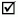# 2Background

Marc Ruiz and Luis Velasco

Universitat Politècnica de Catalunya, Barcelona, Spain

This chapter introduces the basic concepts that are needed to understand the contents of the following chapters. It is organized into five main sections. The first two sections are devoted to graph theory and optimization; basic definitions and notation are presented. The third section illustrates the application of the two previous sections to model a very basic routing problem following several approaches. Basic heuristic methods are introduced, and the two meta‐heuristics commonly used through the book are presented. The last two main sections are devoted to the optical technology and how networks are planned and operated. Section 2.4 introduces the main aspects related to optical networks, starting with an introduction to opaque, translucent, and transparent networks. Then, the key features of Elastic Optical Networks (EONs) are presented. Section 2.5 briefly introduces the classical network life cycle and the essential concepts on connection provisioning and recovery.

 Average Theoretical Practitioner 2.1 Introduction to Graph Theory2.2 Introduction to Optimization 2.3 ILP Models and Heuristics for Routing Problems ...